# Mean State

Period Mean (original grids) [W/m2]
Model Period Mean (intersection) [W/m2]
Model Period Mean (complement) [W/m2]
Benchmark Period Mean (intersection) [W/m2]
Benchmark Period Mean (complement) [W/m2]
Bias [W/m2]
RMSE [W/m2]
Phase Shift [months]
Bias Score 
RMSE Score 
Seasonal Cycle Score 
Spatial Distribution Score 
Interannual Variability Score 
Overall Score 
Benchmark [-] 457.
CRUNCEPv7 [-] 461. 461. 0.00 456. 465. 6.21 15.8 1.44 0.46 0.38 0.79 0.92 0.61 0.59
GSWP3v1 [-] 461. 461. 0.00 456. 465. 5.39 14.8 1.43 0.50 0.39 0.80 1.0 0.60 0.61
WFDEI [-] 460. 460. 0.00 456. 465. 5.42 15.5 1.60 0.52 0.36 0.78 0.98 0.63 0.60
Period Mean (original grids) [W/m2]
Model Period Mean (intersection) [W/m2]
Model Period Mean (complement) [W/m2]
Benchmark Period Mean (intersection) [W/m2]
Benchmark Period Mean (complement) [W/m2]
Bias [W/m2]
RMSE [W/m2]
Phase Shift [months]
Bias Score 
RMSE Score 
Seasonal Cycle Score 
Spatial Distribution Score 
Interannual Variability Score 
Overall Score 
Benchmark [-] 466.
CRUNCEPv7 [-] 481. 481. 0.00 467. 458. 14.5 21.8 0.609 0.52 0.50 0.95 1.0 0.62 0.68
GSWP3v1 [-] 478. 478. 0.00 467. 458. 11.5 19.5 0.566 0.57 0.51 0.95 0.97 0.61 0.69
WFDEI [-] 476. 476. 0.00 467. 458. 9.56 21.3 0.784 0.60 0.46 0.93 1.0 0.66 0.68
Period Mean (original grids) [W/m2]
Model Period Mean (intersection) [W/m2]
Model Period Mean (complement) [W/m2]
Benchmark Period Mean (intersection) [W/m2]
Benchmark Period Mean (complement) [W/m2]
Bias [W/m2]
RMSE [W/m2]
Phase Shift [months]
Bias Score 
RMSE Score 
Seasonal Cycle Score 
Spatial Distribution Score 
Interannual Variability Score 
Overall Score 
Benchmark [-] 443.
CRUNCEPv7 [-] 447. 447. 0.00 443. 446. 4.80 16.5 1.06 0.62 0.51 0.87 1.0 0.61 0.69
GSWP3v1 [-] 447. 447. 0.00 443. 446. 4.28 15.8 1.12 0.67 0.49 0.85 0.99 0.60 0.68
WFDEI [-] 445. 444. 0.00 443. 446. 2.48 17.4 1.50 0.70 0.45 0.79 1.0 0.61 0.67
Period Mean (original grids) [W/m2]
Model Period Mean (intersection) [W/m2]
Model Period Mean (complement) [W/m2]
Benchmark Period Mean (intersection) [W/m2]
Benchmark Period Mean (complement) [W/m2]
Bias [W/m2]
RMSE [W/m2]
Phase Shift [months]
Bias Score 
RMSE Score 
Seasonal Cycle Score 
Spatial Distribution Score 
Interannual Variability Score 
Overall Score 
Benchmark [-] 279.
CRUNCEPv7 [-] 267. 267. 0.00 278. 296. -9.37 18.1 0.135 0.85 0.80 0.99 1.0 0.71 0.86
GSWP3v1 [-] 277. 277. 0.00 278. 296. 2.04 15.4 0.131 0.91 0.81 0.99 1.0 0.74 0.88
WFDEI [-] 261. 261. 0.00 278. 296. -14.9 24.6 0.147 0.79 0.74 0.99 1.0 0.78 0.84
Period Mean (original grids) [W/m2]
Model Period Mean (intersection) [W/m2]
Model Period Mean (complement) [W/m2]
Benchmark Period Mean (intersection) [W/m2]
Benchmark Period Mean (complement) [W/m2]
Bias [W/m2]
RMSE [W/m2]
Phase Shift [months]
Bias Score 
RMSE Score 
Seasonal Cycle Score 
Spatial Distribution Score 
Interannual Variability Score 
Overall Score 
Benchmark [-] 457.
CRUNCEPv7 [-] 455. 455. 0.00 454. 467. 2.62 13.7 1.87 0.31 0.39 0.71 0.85 0.52 0.53
GSWP3v1 [-] 457. 457. 0.00 454. 467. 3.88 14.0 1.70 0.30 0.39 0.73 0.58 0.56 0.49
WFDEI [-] 459. 459. 0.00 454. 467. 6.14 15.1 1.92 0.29 0.36 0.70 0.74 0.65 0.52
Period Mean (original grids) [W/m2]
Model Period Mean (intersection) [W/m2]
Model Period Mean (complement) [W/m2]
Benchmark Period Mean (intersection) [W/m2]
Benchmark Period Mean (complement) [W/m2]
Bias [W/m2]
RMSE [W/m2]
Phase Shift [months]
Bias Score 
RMSE Score 
Seasonal Cycle Score 
Spatial Distribution Score 
Interannual Variability Score 
Overall Score 
Benchmark [-] 312.
CRUNCEPv7 [-] 311. 310. 0.00 311. 334. -0.584 9.49 0.0437 0.94 0.89 1.0 0.99 0.85 0.93
GSWP3v1 [-] 316. 316. 0.00 311. 334. 5.08 11.3 0.0416 0.91 0.89 1.0 1.0 0.86 0.92
WFDEI [-] 306. 306. 0.00 311. 334. -5.31 17.8 0.0327 0.91 0.78 1.0 1.0 0.83 0.88
Period Mean (original grids) [W/m2]
Model Period Mean (intersection) [W/m2]
Model Period Mean (complement) [W/m2]
Benchmark Period Mean (intersection) [W/m2]
Benchmark Period Mean (complement) [W/m2]
Bias [W/m2]
RMSE [W/m2]
Phase Shift [months]
Bias Score 
RMSE Score 
Seasonal Cycle Score 
Spatial Distribution Score 
Interannual Variability Score 
Overall Score 
Benchmark [-] 364.
CRUNCEPv7 [-] 363. 362. 0.00 363. 399. 1.27 13.6 0.117 0.86 0.83 0.99 1.0 0.79 0.88
GSWP3v1 [-] 366. 366. 0.00 363. 399. 4.67 14.4 0.103 0.85 0.83 0.99 0.99 0.75 0.87
WFDEI [-] 359. 359. 0.00 363. 399. -2.13 19.9 0.150 0.83 0.74 0.99 1.0 0.76 0.85
Period Mean (original grids) [W/m2]
Model Period Mean (intersection) [W/m2]
Model Period Mean (complement) [W/m2]
Benchmark Period Mean (intersection) [W/m2]
Benchmark Period Mean (complement) [W/m2]
Bias [W/m2]
RMSE [W/m2]
Phase Shift [months]
Bias Score 
RMSE Score 
Seasonal Cycle Score 
Spatial Distribution Score 
Interannual Variability Score 
Overall Score 
Benchmark [-] 448.
CRUNCEPv7 [-] 455. 456. 0.00 446. 467. 11.2 18.9 0.610 0.56 0.59 0.94 1.0 0.65 0.72
GSWP3v1 [-] 451. 451. 0.00 446. 467. 7.04 17.1 0.677 0.64 0.57 0.93 0.96 0.62 0.72
WFDEI [-] 452. 452. 0.00 446. 467. 8.20 19.9 0.681 0.61 0.52 0.94 0.98 0.64 0.70
Period Mean (original grids) [W/m2]
Model Period Mean (intersection) [W/m2]
Model Period Mean (complement) [W/m2]
Benchmark Period Mean (intersection) [W/m2]
Benchmark Period Mean (complement) [W/m2]
Bias [W/m2]
RMSE [W/m2]
Phase Shift [months]
Bias Score 
RMSE Score 
Seasonal Cycle Score 
Spatial Distribution Score 
Interannual Variability Score 
Overall Score 
Benchmark [-] 281.
CRUNCEPv7 [-] 273. 274. 0.00 281. 288. -6.57 15.1 0.0942 0.89 0.80 0.99 0.98 0.77 0.87
GSWP3v1 [-] 283. 283. 0.00 281. 288. 3.53 13.2 0.0829 0.92 0.82 0.99 1.0 0.76 0.89
WFDEI [-] 268. 269. 0.00 281. 288. -11.6 23.9 0.0929 0.83 0.71 0.99 0.99 0.83 0.84
Period Mean (original grids) [W/m2]
Model Period Mean (intersection) [W/m2]
Model Period Mean (complement) [W/m2]
Benchmark Period Mean (intersection) [W/m2]
Benchmark Period Mean (complement) [W/m2]
Bias [W/m2]
RMSE [W/m2]
Phase Shift [months]
Bias Score 
RMSE Score 
Seasonal Cycle Score 
Spatial Distribution Score 
Interannual Variability Score 
Overall Score 
Benchmark [-] 441.
CRUNCEPv7 [-] 446. 446. 0.00 443. 426. 0.518 12.3 0.380 0.82 0.70 0.97 0.99 0.77 0.83
GSWP3v1 [-] 446. 446. 0.00 443. 426. 0.648 12.3 0.382 0.84 0.70 0.97 1.0 0.72 0.82
WFDEI [-] 444. 444. 0.00 443. 426. -0.660 17.7 0.473 0.80 0.60 0.96 0.99 0.70 0.77
Period Mean (original grids) [W/m2]
Model Period Mean (intersection) [W/m2]
Model Period Mean (complement) [W/m2]
Benchmark Period Mean (intersection) [W/m2]
Benchmark Period Mean (complement) [W/m2]
Bias [W/m2]
RMSE [W/m2]
Phase Shift [months]
Bias Score 
RMSE Score 
Seasonal Cycle Score 
Spatial Distribution Score 
Interannual Variability Score 
Overall Score 
Benchmark [-] 368.
CRUNCEPv7 [-] 363. 363. 0.00 366. 389. -0.518 11.6 0.262 0.85 0.78 0.98 1.0 0.79 0.86
GSWP3v1 [-] 364. 364. 0.00 366. 389. 1.15 11.6 0.230 0.86 0.78 0.98 1.0 0.77 0.86
WFDEI [-] 355. 355. 0.00 366. 389. -7.64 19.2 0.285 0.77 0.65 0.98 0.99 0.82 0.81
Period Mean (original grids) [W/m2]
Model Period Mean (intersection) [W/m2]
Model Period Mean (complement) [W/m2]
Benchmark Period Mean (intersection) [W/m2]
Benchmark Period Mean (complement) [W/m2]
Bias [W/m2]
RMSE [W/m2]
Phase Shift [months]
Bias Score 
RMSE Score 
Seasonal Cycle Score 
Spatial Distribution Score 
Interannual Variability Score 
Overall Score 
Benchmark [-] 399.
CRUNCEPv7 [-] 377. 377. 0.00 376. 409. 2.36 15.5 0.507 0.73 0.68 0.94 1.0 0.68 0.78
GSWP3v1 [-] 380. 380. 0.00 376. 409. 5.23 15.4 0.494 0.74 0.67 0.94 1.0 0.69 0.79
WFDEI [-] 375. 375. 0.00 376. 409. -0.360 18.7 0.603 0.73 0.61 0.93 1.0 0.74 0.77
Period Mean (original grids) [W/m2]
Model Period Mean (intersection) [W/m2]
Model Period Mean (complement) [W/m2]
Benchmark Period Mean (intersection) [W/m2]
Benchmark Period Mean (complement) [W/m2]
Bias [W/m2]
RMSE [W/m2]
Phase Shift [months]
Bias Score 
RMSE Score 
Seasonal Cycle Score 
Spatial Distribution Score 
Interannual Variability Score 
Overall Score 
Benchmark [-] 450.
CRUNCEPv7 [-] 457. 457. 0.00 450. 446. 6.88 16.6 0.344 0.81 0.76 0.97 0.99 0.70 0.83
GSWP3v1 [-] 452. 452. 0.00 450. 446. 2.34 15.0 0.322 0.84 0.76 0.98 1.0 0.71 0.84
WFDEI [-] 447. 447. 0.00 450. 446. -2.71 19.2 0.411 0.83 0.70 0.96 1.0 0.70 0.81
Period Mean (original grids) [W/m2]
Model Period Mean (intersection) [W/m2]
Model Period Mean (complement) [W/m2]
Benchmark Period Mean (intersection) [W/m2]
Benchmark Period Mean (complement) [W/m2]
Bias [W/m2]
RMSE [W/m2]
Phase Shift [months]
Bias Score 
RMSE Score 
Seasonal Cycle Score 
Spatial Distribution Score 
Interannual Variability Score 
Overall Score 
Benchmark [-] 281.
CRUNCEPv7 [-] 278. 278. 0.00 281. 293. -3.57 15.7 0.202 0.90 0.79 0.98 0.99 0.70 0.86
GSWP3v1 [-] 287. 287. 0.00 281. 293. 6.28 16.8 0.170 0.89 0.79 0.99 1.0 0.76 0.87
WFDEI [-] 273. 273. 0.00 281. 293. -8.10 21.9 0.193 0.86 0.72 0.98 0.98 0.83 0.85
Period Mean (original grids) [W/m2]
Model Period Mean (intersection) [W/m2]
Model Period Mean (complement) [W/m2]
Benchmark Period Mean (intersection) [W/m2]
Benchmark Period Mean (complement) [W/m2]
Bias [W/m2]
RMSE [W/m2]
Phase Shift [months]
Bias Score 
RMSE Score 
Seasonal Cycle Score 
Spatial Distribution Score 
Interannual Variability Score 
Overall Score 
Benchmark [-] 442.
CRUNCEPv7 [-] 443. 443. 0.00 439. 459. 8.32 16.8 0.816 0.56 0.56 0.90 1.0 0.68 0.71
GSWP3v1 [-] 444. 444. 0.00 439. 459. 8.98 16.4 0.854 0.56 0.57 0.89 0.96 0.67 0.70
WFDEI [-] 445. 445. 0.00 439. 459. 10.1 19.1 0.916 0.54 0.51 0.89 0.98 0.72 0.69
Period Mean (original grids) [W/m2]
Model Period Mean (intersection) [W/m2]
Model Period Mean (complement) [W/m2]
Benchmark Period Mean (intersection) [W/m2]
Benchmark Period Mean (complement) [W/m2]
Bias [W/m2]
RMSE [W/m2]
Phase Shift [months]
Bias Score 
RMSE Score 
Seasonal Cycle Score 
Spatial Distribution Score 
Interannual Variability Score 
Overall Score 
Benchmark [-] 386.
CRUNCEPv7 [-] 388. 388. 0.00 384. 429. 6.21 11.1 0.106 0.86 0.86 0.99 0.99 0.87 0.90
GSWP3v1 [-] 390. 390. 0.00 384. 429. 7.99 11.9 0.0700 0.84 0.86 1.0 1.0 0.85 0.90
WFDEI [-] 383. 383. 0.00 384. 429. 1.09 16.4 0.0844 0.89 0.73 0.99 0.99 0.90 0.87
Period Mean (original grids) [W/m2]
Model Period Mean (intersection) [W/m2]
Model Period Mean (complement) [W/m2]
Benchmark Period Mean (intersection) [W/m2]
Benchmark Period Mean (complement) [W/m2]
Bias [W/m2]
RMSE [W/m2]
Phase Shift [months]
Bias Score 
RMSE Score 
Seasonal Cycle Score 
Spatial Distribution Score 
Interannual Variability Score 
Overall Score 
Benchmark [-] 324.
CRUNCEPv7 [-] 324. 324. 0.00 323. 347. 1.68 10.7 0.0546 0.91 0.84 1.0 0.99 0.78 0.89
GSWP3v1 [-] 329. 329. 0.00 323. 347. 6.71 13.5 0.0540 0.87 0.83 1.0 0.97 0.78 0.88
WFDEI [-] 320. 320. 0.00 323. 347. -2.43 18.4 0.0791 0.91 0.73 0.99 0.99 0.84 0.87
Period Mean (original grids) [W/m2]
Model Period Mean (intersection) [W/m2]
Model Period Mean (complement) [W/m2]
Benchmark Period Mean (intersection) [W/m2]
Benchmark Period Mean (complement) [W/m2]
Bias [W/m2]
RMSE [W/m2]
Phase Shift [months]
Bias Score 
RMSE Score 
Seasonal Cycle Score 
Spatial Distribution Score 
Interannual Variability Score 
Overall Score 
Benchmark [-] 377.
CRUNCEPv7 [-] 377. 378. 0.00 377. 378. 1.16 13.8 0.0965 0.84 0.82 0.99 0.99 0.78 0.87
GSWP3v1 [-] 380. 381. 0.00 377. 378. 4.28 13.6 0.0956 0.85 0.82 0.99 1.0 0.73 0.87
WFDEI [-] 376. 377. 0.00 377. 378. 0.492 19.3 0.126 0.86 0.71 0.99 0.99 0.82 0.85
Period Mean (original grids) [W/m2]
Model Period Mean (intersection) [W/m2]
Model Period Mean (complement) [W/m2]
Benchmark Period Mean (intersection) [W/m2]
Benchmark Period Mean (complement) [W/m2]
Bias [W/m2]
RMSE [W/m2]
Phase Shift [months]
Bias Score 
RMSE Score 
Seasonal Cycle Score 
Spatial Distribution Score 
Interannual Variability Score 
Overall Score 
Benchmark [-] 449.
CRUNCEPv7 [-] 457. 457. 0.00 449. 463. 7.89 14.1 1.11 0.34 0.41 0.85 0.96 0.66 0.61
GSWP3v1 [-] 457. 457. 0.00 449. 463. 8.33 14.2 1.22 0.34 0.40 0.84 1.0 0.62 0.60
WFDEI [-] 457. 457. 0.00 449. 463. 8.68 15.3 1.76 0.35 0.34 0.74 0.99 0.69 0.57
Period Mean (original grids) [W/m2]
Model Period Mean (intersection) [W/m2]
Model Period Mean (complement) [W/m2]
Benchmark Period Mean (intersection) [W/m2]
Benchmark Period Mean (complement) [W/m2]
Bias [W/m2]
RMSE [W/m2]
Phase Shift [months]
Bias Score 
RMSE Score 
Seasonal Cycle Score 
Spatial Distribution Score 
Interannual Variability Score 
Overall Score 
Benchmark [-] 443.
CRUNCEPv7 [-] 447. 447. 0.00 443. 434. 3.42 12.5 0.714 0.74 0.59 0.90 1.0 0.67 0.75
GSWP3v1 [-] 447. 447. 0.00 443. 434. 3.23 12.7 0.810 0.76 0.57 0.89 1.0 0.65 0.74
WFDEI [-] 443. 443. 0.00 443. 434. -0.558 15.5 1.00 0.76 0.49 0.86 1.0 0.68 0.71
Period Mean (original grids) [W/m2]
Model Period Mean (intersection) [W/m2]
Model Period Mean (complement) [W/m2]
Benchmark Period Mean (intersection) [W/m2]
Benchmark Period Mean (complement) [W/m2]
Bias [W/m2]
RMSE [W/m2]
Phase Shift [months]
Bias Score 
RMSE Score 
Seasonal Cycle Score 
Spatial Distribution Score 
Interannual Variability Score 
Overall Score 
Benchmark [-] 383.
CRUNCEPv7 [-] 383. 383. 0.00 381. 396. 2.29 19.9 1.02 0.64 0.57 0.87 1.0 0.63 0.71
GSWP3v1 [-] 388. 387. 0.00 381. 396. 6.19 20.3 0.938 0.62 0.57 0.88 0.99 0.62 0.71
WFDEI [-] 389. 389. 0.00 381. 396. 8.00 22.6 0.945 0.61 0.51 0.88 1.0 0.67 0.70

# Temporally integrated period mean

BENCHMARK MEAN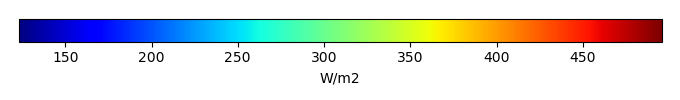MODEL MEANBIAS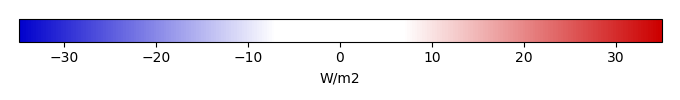BIAS SCORERMSE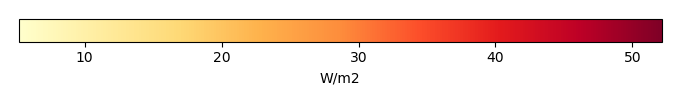RMSE SCOREBENCHMARK INTERANNUAL VARIABILITY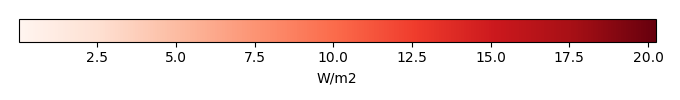MODEL INTERANNUAL VARIABILITYINTERANNUAL VARIABILITY SCOREBENCHMARK MAX MONTHMODEL MAX MONTHDIFFERENCE IN MAX MONTH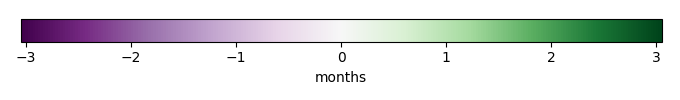SEASONAL CYCLE SCORESPATIAL TAYLOR DIAGRAMMODEL COLORS# Spatially integrated regional mean

MODEL COLORSREGIONAL MEANANNUAL CYCLEMONTHLY ANOMALYANNUAL CYCLE# All Models

BenchmarkCRUNCEPv7GSWP3v1WFDEI# Data Information

creation_date: Thu May 8 23:13:11 PDT 2014

source_file: This product is generated from monthly 1 degree CERES EBAF Radiation observations